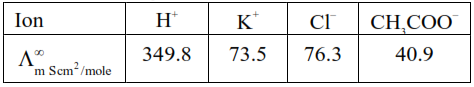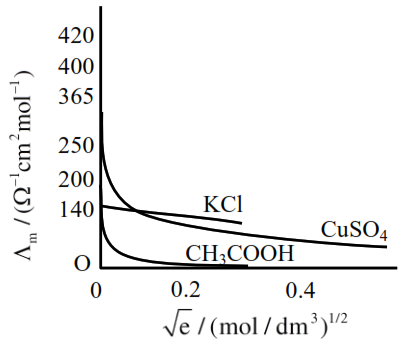# Given below are two statements :`
Question:

Given below are two statements :

Statement I : The limiting molar conductivity of $\mathrm{KCl}$ (strong electrolyte) is higher compared to that of $\mathrm{CH}_{3} \mathrm{COOH}$ (weak electrolyte).

Statement II : Molar conductivity decreases with decrease in concentration of electrolyte.

In the light of the above statements, choose the most appropriate answer from the options given below:

1. Statement I is true but Statement II is false.

2. Statement I is false but Statement II is true.

3. Both Statement I and Statement II are true.

4. Both Statement I and Statement II are false.

Correct Option: , 4

Solution:So $\Lambda_{\mathrm{m} \mathrm{CH}_{3} \mathrm{COOH}}^{\infty}=\Lambda_{\mathrm{m}\left(\mathrm{H}^{+}\right)}^{\infty}+\Lambda_{\mathrm{m} \mathrm{CH}_{3} \mathrm{COO}^{-}}^{\infty}$

$=349.8+40.9$

$=390.7 \mathrm{Scm}^{2} / \mathrm{mole}$

$\Lambda_{\mathrm{m} \mathrm{KCl}}^{\infty}=\Lambda_{\mathrm{m}\left(\mathrm{K}^{+}\right)}^{\infty}+\Lambda_{\mathrm{m}\left(\mathrm{Cl}^{-}\right)}^{\infty}$

$=73.5+76.3$

$=149.3 \mathrm{Scm}^{2} / \mathrm{mole}$

So statement-I is wrong or False.

As the concentration decreases, the dilution increases which increases the degree of dissociation, thus increasing the no. of ions, which increases the molar conductance.

So statement-II is false.